# Consider two \$60,000 investments – call them Investment A and Investment B. Both investments will...

Consider two \$60,000 investments – call them Investment A and Investment B. Both investments will earn \$5,000 with a probability of 0.5 and \$1,000 with a probability of 0.5. Investment A will use 100% equity financing (issuing stocks). Investment B will get \$30,000 through issuing stocks and \$30,000 through issuing bonds. Investment B must pay 4% interest on the bonds.

a. Calculate the expected returns on equity (returns after interest payments divided by the amount of equity) for Investment A and Investment B. Express the returns as a percentage.

b. If the investments earned the lower amount (\$1,000), what is the rate of return on equity for Investment A and Investment B? If the investments earned the higher amount (\$5,000), what is the return on equity for Investment A and Investment B?

c. Using your answers from ‘a’ and ‘b’, what is the standard deviation of the rate of return on equity in each case? Which investment has the highest expected returns on equity? Which has the lowest risk? What explains the difference in risk between the two investments?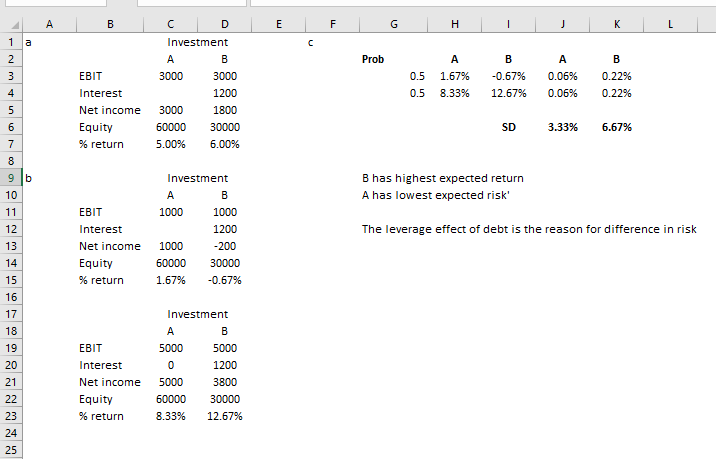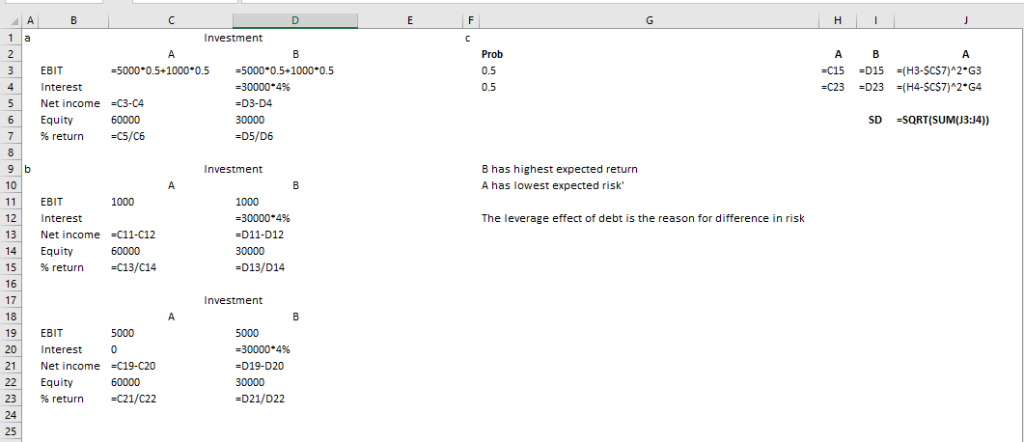##### Add Answer of: Consider two \$60,000 investments – call them Investment A and Investment B. Both investments will...
Similar Homework Help Questions
• ### SLIDII 2 Suppose that you are investigating two mutual exclusive investments (investment A and B) which...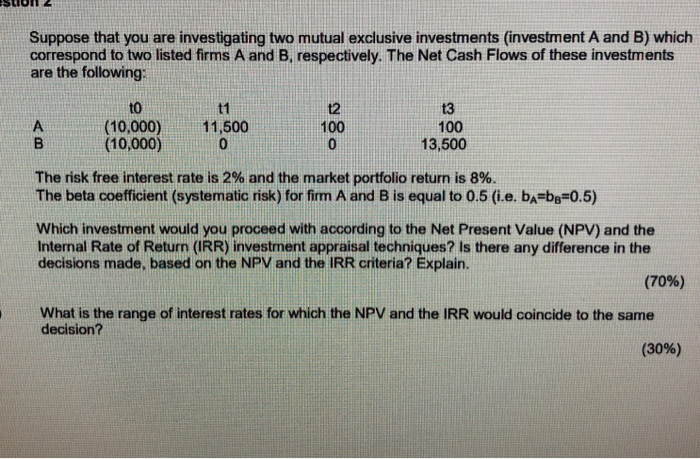SLIDII 2 Suppose that you are investigating two mutual exclusive investments (investment A and B) which correspond to two listed firms A and B, respectively. The Net Cash Flows of these investments are the following: 13 100 (10,000) (10,000) 11,500 0 ogs B 13,500 The risk free interest rate is 2% and the market portfolio return is 8%. The beta coefficient (systematic risk) for firm A and B is equal to 0.5 (i.e. ba=bg=0.5) Which investment would you proceed with...

• ### Consider the following two investments. One is a risk-free investment with a \$100 return. The oth...

Consider the following two investments. One is a risk-free investment with a \$100 return. The other investment pays \$2,000 20% of the time and a \$375 loss the rest of the time. Based on this information, answer the following: (i) Compute the expected returns and standard deviations on these two investments individually. (ii) Compute the value at risk for each investment. (iii) Which investment will risk-averse investors prefer, if either? Which investment will risk- neutral investors prefer, if either?

• ### An investor is considering three types of investments: a high-risk venture into oil leases with a potential return of 15%, a medium-risk investment in stocks with 9% return, and a relatively safe bond investment with a 5% return

An investor is considering three types of investments: a high-risk venture into oil leases with a potential return of 15%, a medium-risk investment in stocks with 9% return, and a relatively safe bond investment with a 5% return. He had \$50,000 to invest. Because of the risk, he will limit his investment in oil leases and stocks to 30% and his investment in oil leases and bonds to 50%. How much should he invest in each to maximize his return,...

• ### Investment Portfolio You are an investment manager for Simple Asset Management, a company that specializes in...Investment Portfolio You are an investment manager for Simple Asset Management, a company that specializes in developing simple investment portfolios consisting of no more than three assets such as stocks, bonds, etc., for investors who like to keep things simple. One of your more popular investments is called the All World Fund and is composed of global stocks with good dividend yields. A client is interested in constructing a portfolio that consists of the All World Fund and the Treasury...

• ### Consider the following expected return on two stocks for two particular market returns: With probability 1/2...

Consider the following expected return on two stocks for two particular market returns: With probability 1/2 the market return is equal to 4%, return of stock A is 1% and B is 6%. With probability 1/2 the market return is equal to 20%, return of stock A is 33% and B is 10%. (Hint: these are realizations and not expected values, you should calculate the expected returns using the given probabilities and returns) (a) What is the expected rate of...

• ### Suppose that you investigate the case of two mutual exclusive investments, investment A and investment B,...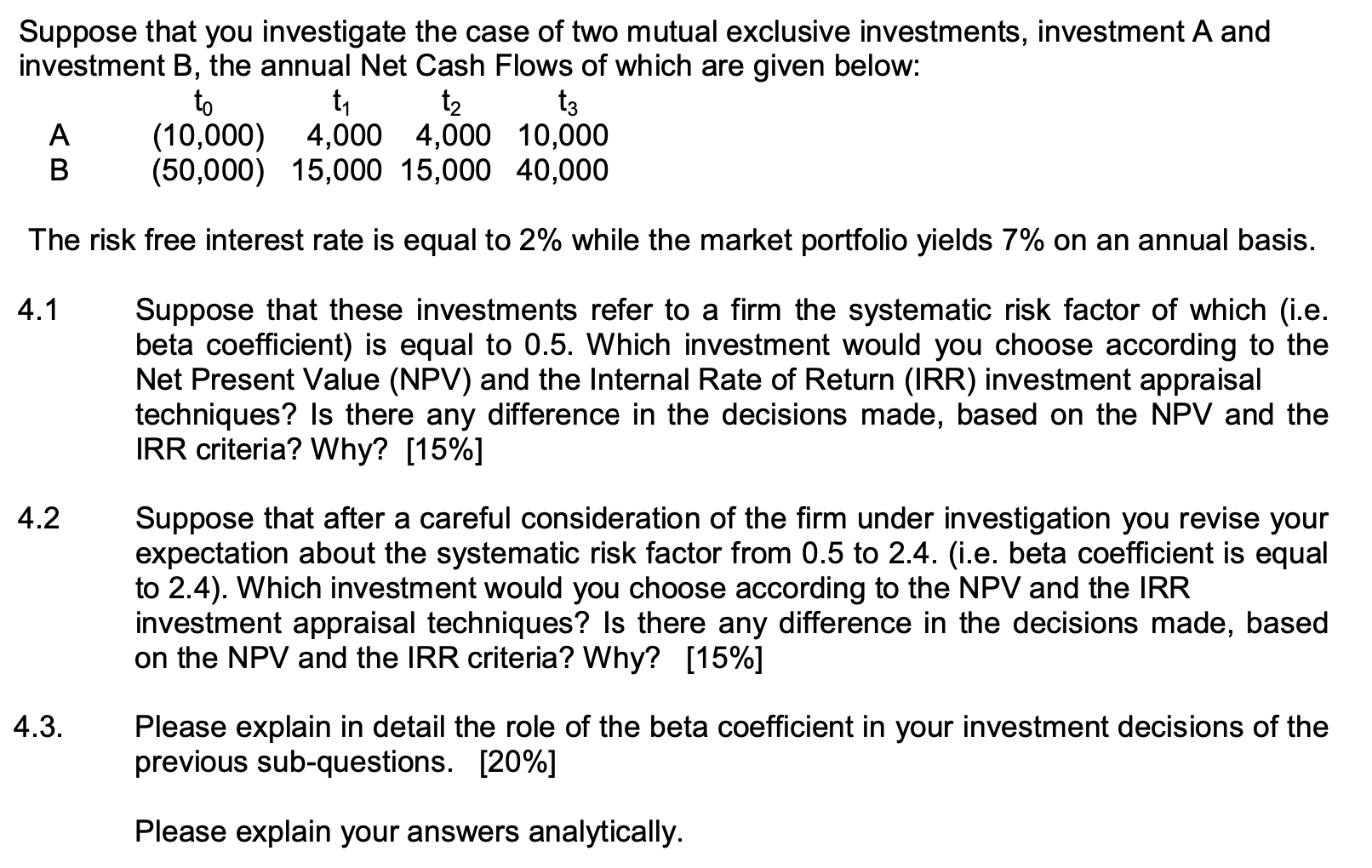Suppose that you investigate the case of two mutual exclusive investments, investment A and investment B, the annual Net Cash Flows of which are given below: to ti tz tz A (10,000) 4,000 4,000 10,000 B (50,000) 15,000 15,000 40,000 The risk free interest rate is equal to 2% while the market portfolio yields 7% on an annual basis. 4.1 Suppose that these investments refer to a firm the systematic risk factor of which (i.e. beta coefficient) is equal to...

• ### 2. Consider the following expected return on two stocks for two particular market returns: With probability...

2. Consider the following expected return on two stocks for two particular market returns: With probability 1/2 the market return is equal to 4%, return of stock A is 1% and B is 6%. With probability 1/2 the market return is equal to 20%, return of stock A is 33% and B is 10%. (Hint: these are realizations and not expected values, you should calculate the expected returns using the given probabilities and returns) (a) What is the expected rate...

• ### Investment Portfolio You are an investment manager for Simple Asset Management, a company that specializes in...

Investment Portfolio You are an investment manager for Simple Asset Management, a company that specializes in developing simple investment portfolios consisting of no more than three assets such as stocks, bonds, etc., for investors who like to keep things simple. One of your more popular investments is called the All World Fund and is composed of global stocks with good dividend yields. A client is interested in constructing a portfolio that consists of the All World Fund and the Treasury...

• ### 5. Assume that an investment is forecasted to produce the following returns: a 20% probability of...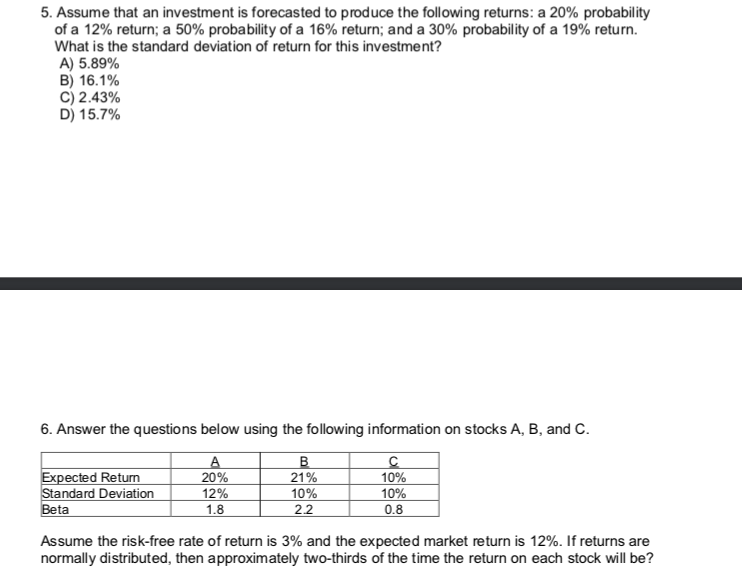5. Assume that an investment is forecasted to produce the following returns: a 20% probability of a 12% return; a 50% probability of a 16% return; and a 30% probability of a 19% return. What is the standard deviation of return for this investment? A) 5.89% B) 16.1% C) 2.43% D) 15.7% 6. Answer the questions below using the following information on stocks A, B, and C. Expected Return Standard Deviation Beta 20% 12% 1.8 21% 10% 2.2 10% 10%...

• ### An investment amount of \$10M has to be raised through equity financing and debt financing. The...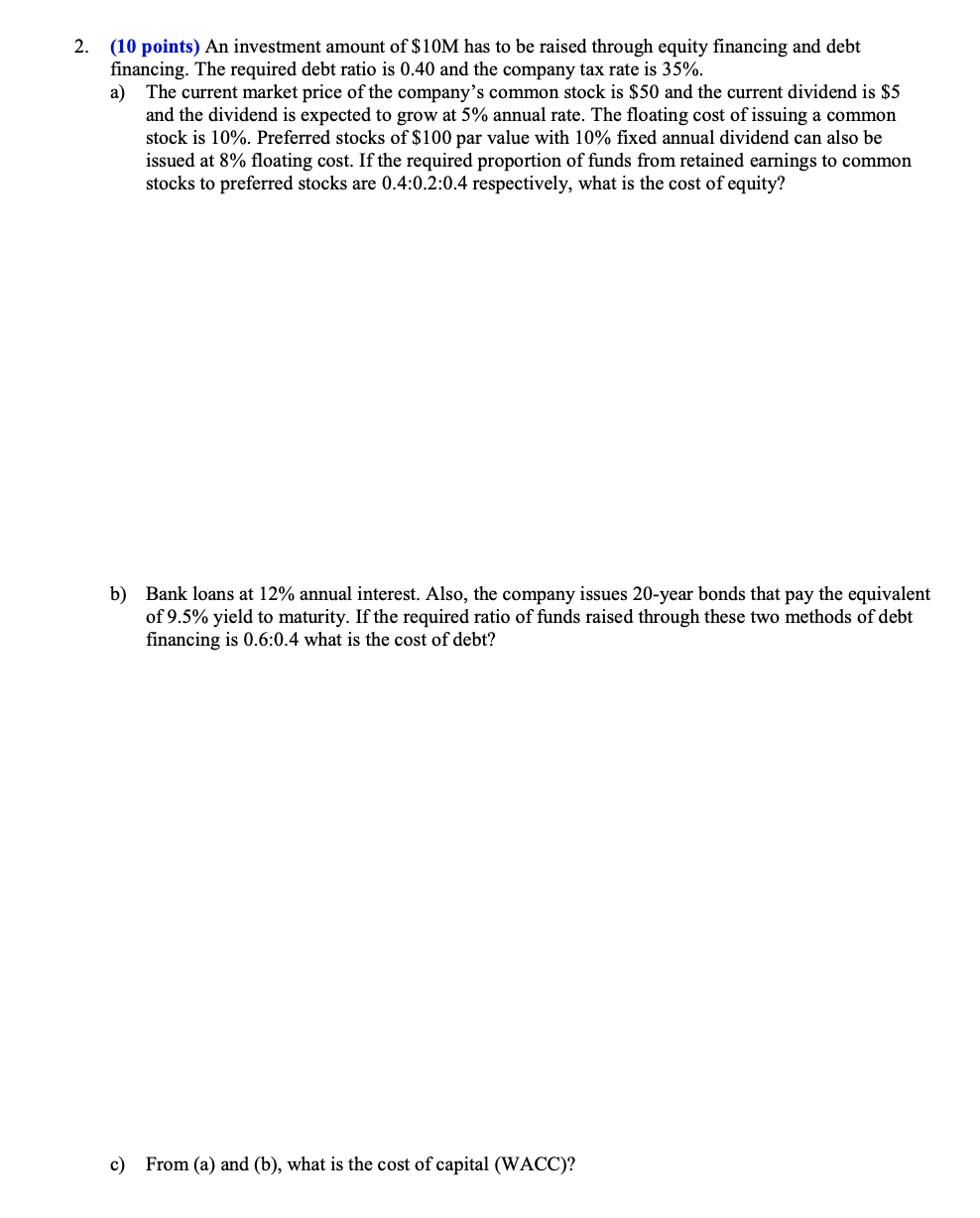An investment amount of \$10M has to be raised through equity financing and debt financing. The required debt ratio is 0.40 and the company tax rate is 35%. a) The current market price of the company’s common stock is \$50 and the current dividend is \$5 and the dividend is expected to grow at 5% annual rate. The floating cost of issuing a common stock is 10%. Preferred stocks of \$100 par value with 10% fixed annual dividend can also...

Need Online Homework Help?